Understanding Zero-Knowledge Proofs in 15 Mins through SNARK and STARK

## Background

If you have read my article on Ring-Trade Systems (check it out here), you know that I ended my argument on one assumption — A way to securely roll-up computationally intensive operations like ring-matching from L2 to L1. In the case of Loopring, the solution was a ZK roll-up. While I will save the Optimistic vs. ZK conversation to a potential future article, I do want to take some time to explain ZK, whose cameo in most articles consists of "…without getting into the mathematics…" and ".. it's basically magic…"

## The Problem — Trust through computation

When you need someone else to do something for you, such as trimming the lawn, transferring your money, or casting a vote, how do you ensure they will do what they promise to do? Granted, you can probably watch Mike, the 9-year-old pushing that manual lawnmower down your front lawn as you enjoy your Lipton Tea next to the window; there are too many black-boxes today that you simply cannot verify the work of all the things we can't perform ourselves.That's where trust comes in. You would trust a national bank that sponsored your city's hockey stadium to be responsible with your money and information(or not. Hello Wells Fargo), but would you trust some website that takes your real money and gives something called Magical Internet Money in return? (learn more here)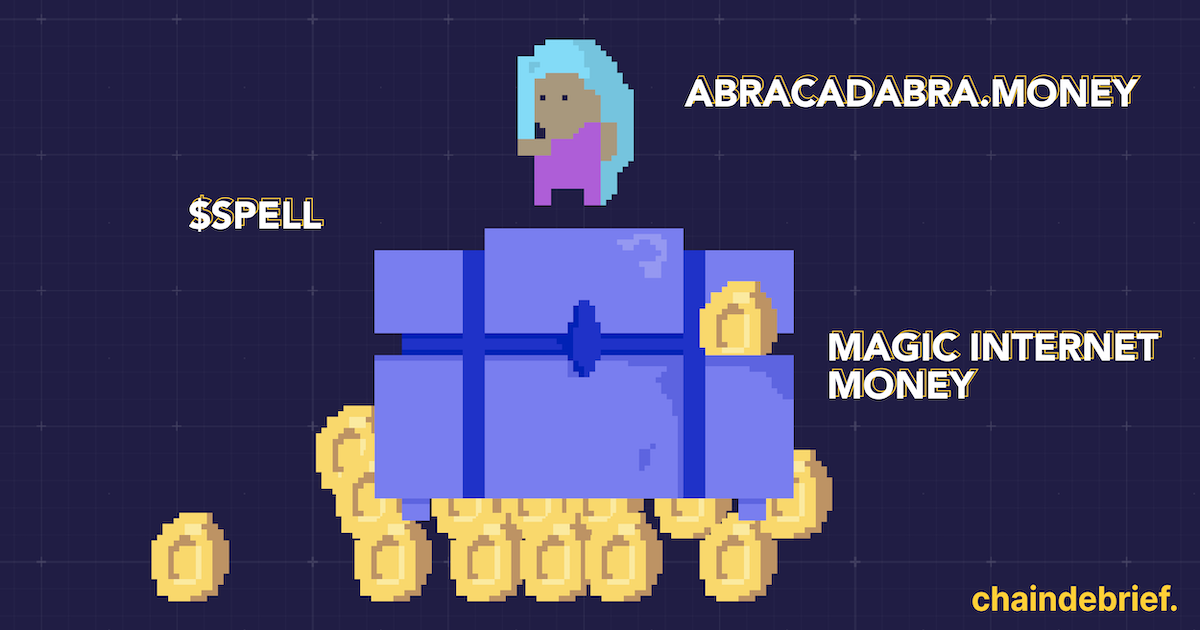The lack of trust is such a big problem-space in our daily life — politics, food, environment… So let's narrow it down — how can you trust a computer to execute a program truthfully?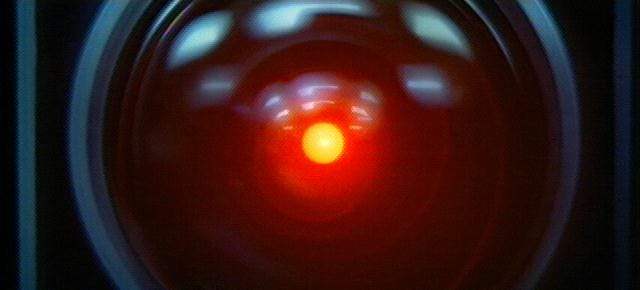Or they are straight-up evil

One way to do this is simply to have a sufficient number of independent "verifiers" to "rerun" the program and see whether the results align. Let's say that your friend Mike says that 2+2 = 4. If 10 other of your friends also got 4 as the answer to "2+2", Mike most likely did a good job. If you want, you can even do the addition yourself and be certain that the answer is correct.The above approach to verify an answer or achieve "consensus" is precisely how blockchains like Ethereum ensure its security — by essentially simple majority (with some fancy cryptography ofc.) However, since every computation needs to be replicated and verified by all participants (a part of the mining process), even a five-year-old can see why this can be inefficient.

More importantly, getting other miners to "work for you" or run computations to do something you want (convert tokens, send funds, mint NFTs, etc.) is expensive. The inefficiency translates to high gas fees(think tips) you need to pay for the blockchain's consensus.Another issue of the naive "rerun" verification is the lack of privacy. In many applications, you want privacy in the programs you wish to run. Perhaps it contains a porn subscription or privileged financial data that you don't want everyone in the world to know about. In that case, a transparent, consensus-driven computer would do no good to protect your privacy.If only there exists a way for computers to prove that they did each step of the computation truthfully (or CI, computation integrity), without revealing the privileged data, fast, and can be verified by a secure blockchain network…

The Set-Up

Our goal is to have A (the Prover) execute a computer program given some inputs to be kept private. Then, A produces a special Proof that can convince B (the Verifier) that the program is executed correctly without revealing the private inputs or the result, and only taking little computation.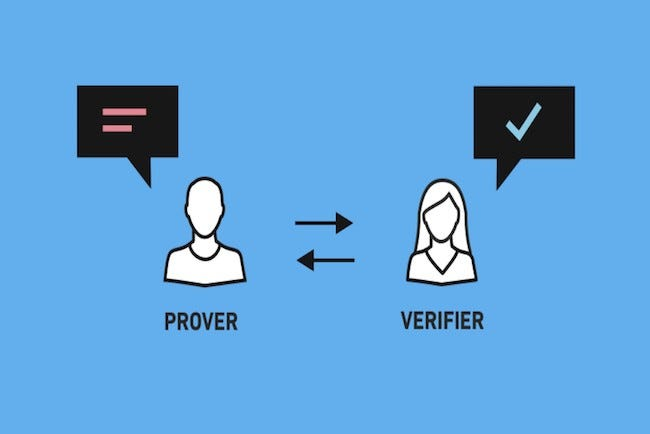## Introducing ZKP

Zero-Knowledge Proof is such a class of systems to do exactly what we want above. Popular implementations include SNARK and STARK, each with unique attributes.

zk-SNARK: zero-knowledge Succinct Non-Interactive Argument of Knowledge

zk-STARK: zero-knowledge Scalable Transparent ARgument of Knowledge

Today, ZKP is often used to verify the integrity of expensive operations performed off of the public blockchain, thus providing a performant and efficient alternative to handling everything on the public blockchain.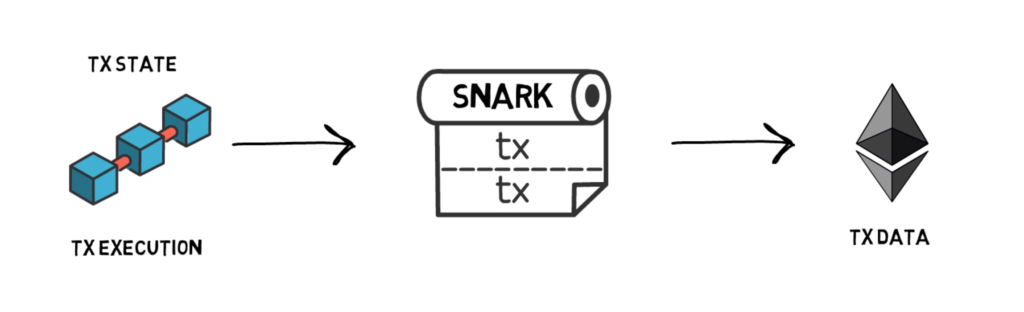A typical workflow of a ZKP looks like this:

1. Some pre-determined program is set to be performed on an off-chain computer.
2. The program executes, yielding some results.
3. In addition to the program itself, the computer runs a Prover program, which generates Proof that certifies of the code executing correctly.
4. The Proof is sent back to the blockchain, containing no private data.
5. A Verifier smart contract runs with the Proof as the input. It either accepts or rejects the off-chain program is run correctly with very high certainty.
6. The result of the program is either accepted or rejected

Now you must have a question —** BUT HOW!**On a high level, ZKP leverages a series of "if A then B," "if B then C,"… which ultimately yields "if Proof is verified, then the computation is performed correctly" without revealing any sensitive information. More concretely, we need a series of transformations to convert a program to a mathematical statement, at which point we can apply further math and cryptography magic to turn it into a ZKP.

In the below steps, I will explain each of the stages, as well as how SNARK and STARK handle some stages differently.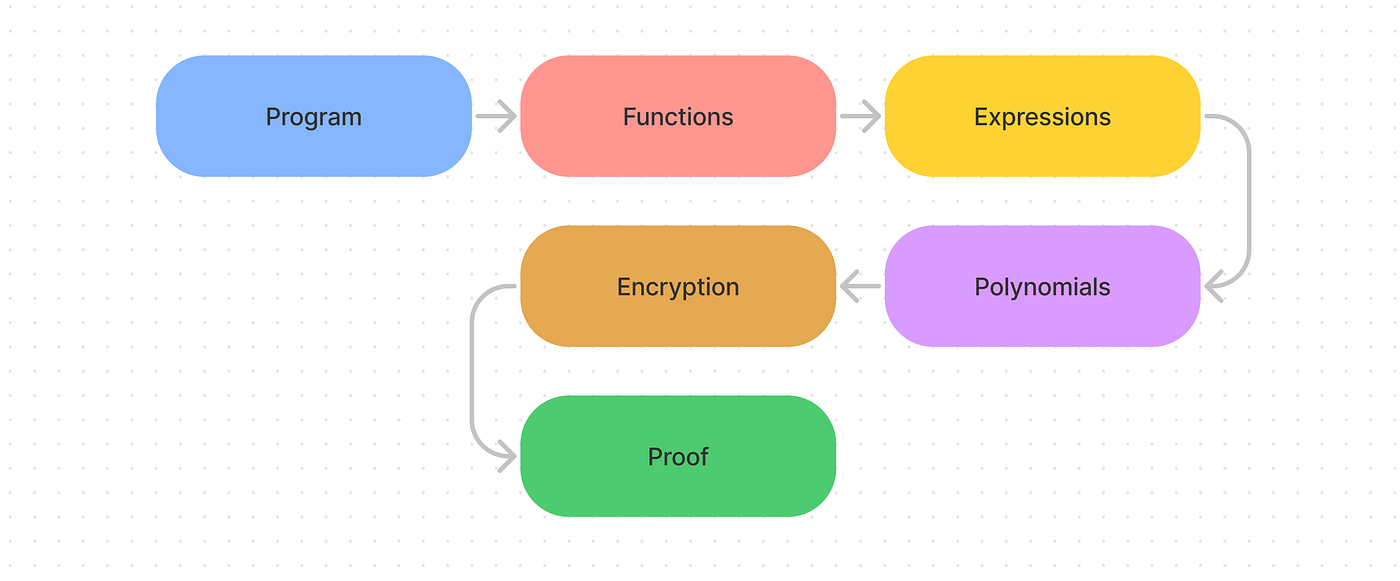## 1. Program->functions

By definition, a program consists of a series of functions invoked in some set orders. It's easy to see that if we can prove the underlying functions, then the program should, in turn, be proven to perform "correctly". Alternatively, a program can be considered a mega-function that contains one or multiple functions. For the purpose of this article, we'll explain ZKP on a function level.

## **2. Function -> **mathematical expressions

Writing any function in code consists of a series of operations. Each operation can be as complex as something like a For-Loop, or something as trivial as x=x+1. Regardless, all computer does ultimately is flip bits. Thus, it's trivial to see operations as some mathematical relationships, and a function as a system of equations.On a high level, both methods turn the function into a mathematical expression that is easier to work with. SNARK uses R1CS (Rank-1 Constraint System) as its mathematical encoding, and STARK uses AIR (Algebraic Intermediate Representation).

## SNARK

A function like below:

``````def qeval(x):
y = x**3
return x + y + 5
``````

can be flattened into simple expressions like these, satisfying the form `x=y` and `x=y(operator)z`:

``````sym_1 = x * x
y = sym_1 * x
sym_2 = y + x
~out = sym_2 + 5
``````

In this simple case, we have very limited Mathematics lingo (* and +). However, with the help of some additional math constructs (binary circuits, finite field arithmetic, etc.,) it's possible to simulate all the basic gates (from which you can build everything a computer needs). To keep the example simple, we'll stick with the naive construction.

Conversion to R1CS

In our example, we will proceed to convert the above-flattened function into a Rank-1 Constraint System which maintains the form of `s.a * s.b — s.c = 0` , where `s` is the solution, and `a,b,c` encoded below

``````A
[0, 1, 0, 0, 0, 0]
[0, 0, 0, 1, 0, 0]
[0, 1, 0, 0, 1, 0]
[5, 0, 0, 0, 0, 1]B
[0, 1, 0, 0, 0, 0]
[0, 1, 0, 0, 0, 0]
[1, 0, 0, 0, 0, 0]
[1, 0, 0, 0, 0, 0]C
[0, 0, 0, 1, 0, 0]
[0, 0, 0, 0, 1, 0]
[0, 0, 0, 0, 0, 1]
[0, 0, 1, 0, 0, 0]
``````

the vector assignment is

``````'~one', 'x', '~out', 'sym_1', 'y', 'sym_2'
``````

If you don't quite understand this transformation, just trust me (as you will have to for the rest of the article) that this does indeed encode the simple function above.

## STARK

On the other hand, STARK cares not only about the constraints that encode the function but also the step-by-step trace of the function run.

Take the example of a Collatz Sequence; we first have the constraints that define the function(1)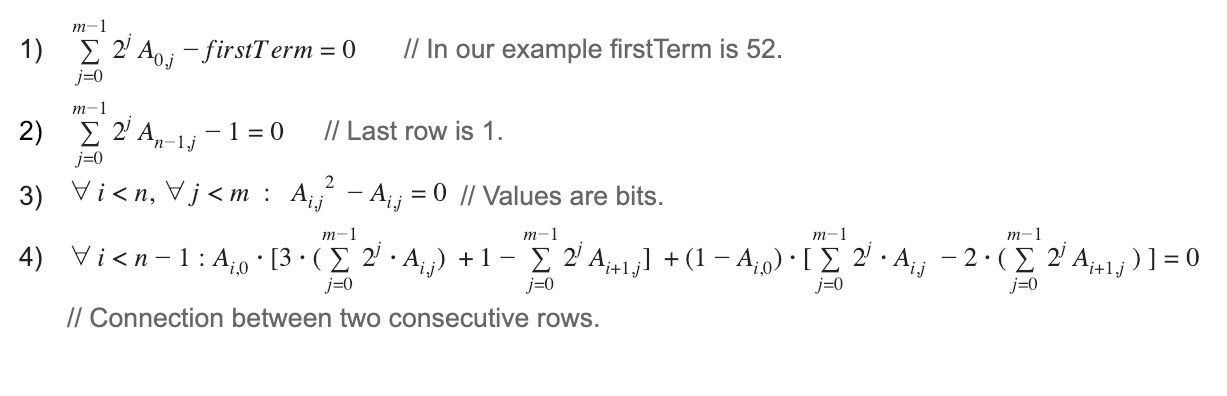But also the execution trace of the sequence(2)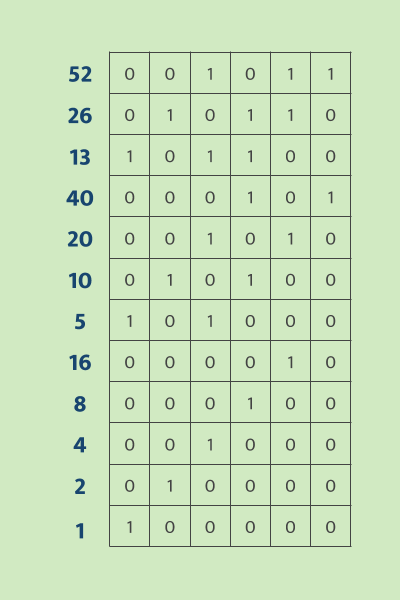blue is the decimal number, and the grid is binary(MSB on the right)

If the computation is performed truthfully, then every step should align with the constraints above.

The collection of the above constraints (to be turned into polynomials next) and the execution trace becomes what STARK calls AIR (Algebraic Intermediate Representation), which is the succinct representation of the mathematical problem the ZK proof is trying to verify,

## 4. Expressions -> Polynomials

It turns out that the above expressions are still not something that can be used directly. Instead, the R1CSs and AIRs need to be turned into some polynomial that can be evaluated.

In this step, SNARK takes a more direct approach where the solution to the problem is (for now) the solution to the resulting polynomial. On the other hand, STARK turns the two ingredients of its AIR into an intermediate problem in preparation for the next step.

## SNARK

Since SNARK is only evaluating the function itself, the next step in the process involves encoding the R1CS into polynomials, and particularly, the form of QAP(Quadratic Arithmetic Programs).

First, we use Lagrange Interpolation (turns points into a curve) to interpolate polynomials that encodes our points from above when evaluated at different inputs

``````A polynomials
[-5.0, 9.166, -5.0, 0.833]
[8.0, -11.333, 5.0, -0.666]
[0.0, 0.0, 0.0, 0.0]
[-6.0, 9.5, -4.0, 0.5]
[4.0, -7.0, 3.5, -0.5]
[-1.0, 1.833, -1.0, 0.166]B polynomials
[3.0, -5.166, 2.5, -0.333]
[-2.0, 5.166, -2.5, 0.333]
[0.0, 0.0, 0.0, 0.0]
[0.0, 0.0, 0.0, 0.0]
[0.0, 0.0, 0.0, 0.0]
[0.0, 0.0, 0.0, 0.0]C polynomials
[0.0, 0.0, 0.0, 0.0]
[0.0, 0.0, 0.0, 0.0]
[-1.0, 1.833, -1.0, 0.166]
[4.0, -4.333, 1.5, -0.166]
[-6.0, 9.5, -4.0, 0.5]
[4.0, -7.0, 3.5, -0.5]
``````

If you plug in 1 into the A polynomials (ascending coefficients), you would get the first vector from the A vectors in the step above

Now we have the R1CS/QAP below to check validity.In this case `[1,3,35,9,27,30]` is a valid set of input (witness) of our previous encoding that would satisfy the R1CS. To evaluate this equation, rather than evaluating the solution at every point, we can take a shortcut by verifying the left side `t` when dividing `Z` (defined as `(x-1)*(x-2)*(x-3)...`) gives no remainder.

## STARK

There are two "ingredients" (from AIR) that needs to turn into polynomials for STARK

1. Constraint system encodes the calculation
2. Execution trace values

The challenge for the former is ultimately about cleanly describing a piece of code, algorithm… into a concise and efficiently constructed polynomial that encodes the problem (Ideally with some recursive component). While this may seem trivial for something like a Fibonacci Sequence, the same can't be said for other more complex mechanisms.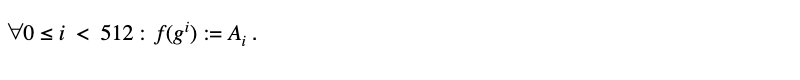Fibonacci polynomial

As for the execution trace, STARK encodes the value of each step into a polynomial `f`such that for some generator `g` , `f(g^i)` is `A_i` which is the value of the `i-th` step of the trace.Traced "steps" of Fibonacci

This can be done by using something like Lagrange Interpolation and resulting in a polynomial with at most degree `d` .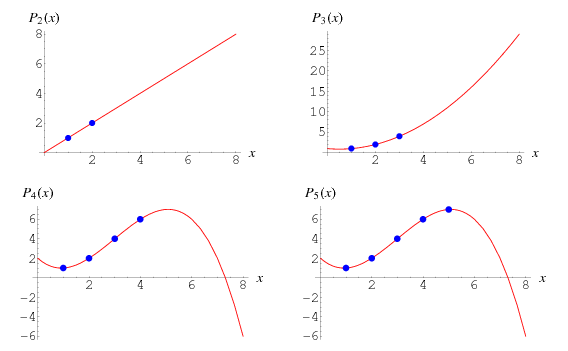Lagrange Interpolation Illustration

## 5. Interlude: Polynomials -> Encrypted Polynomials

Up to this point, we have yet to encrypt our input (the zero-knowledge part). To satisfy the "zero-knowledge" requirement, SNARK and STARK again diverge in their approaches.SNARK bases its encryption on private-public key encryption, essentially showing you some rooms, each with its door locked so you can't see what's inside.STARK, however, uses Shamir's Secret Sharing Scheme which is like a treasure hunt that omits the last challenge that points to the treasure. Without completing the last challenge, the treasure is always safe.

## SNARK

SNARKs use a generic scheme of encryption algorithms — An encryption algorithm that transforms input to something else based on a special encryption function. The encryption function has one or a set of parameters that is its key. Just like a door, the key enables its owner to easily lock and unlock the encrypted object (called a "trapdoor function"). Conversely, unlocking without a key would be much much more difficult.Trapdoor

To encrypt in SNARK, we use an encryption algorithm called Elliptic Curve Cryptography or ECC.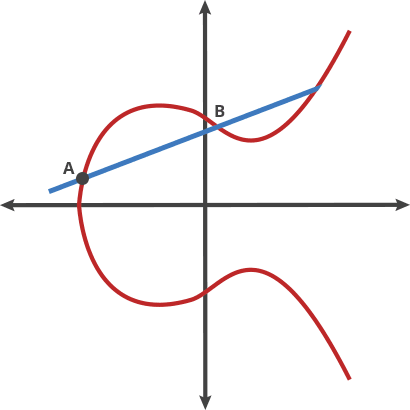Essentially, ECC can take an input `P` and apply a specially defined "dot" function on itself for `n`times and arrive at `Q`. What's special about this process is that given `P`and `Q`, it's non-trivial to find the encryption key `n`, however, given `P` and `n` , it's quite easy to find `Q`, clearly a "trapdoor function"

The above paragraph clearly highlights the importance of the encryption key. If the key is simply given to the Prover of the message, then it can easily fake a proof. We can't trust any computer to act non-maliciously, especially the Prover, who we must assume would try to cheat at any opportunity. Luckily, the mathematical property of ECC allowed the key itself to be wrapped in a function while still being able to be used.

These "essence" parameters (wrapped key) are created in a "trusted set-up" with the actual key securely discarded, leaving only the "essence" which is cryptographically tamper-proof to encrypt the computations.

An example of a trusted set-up

We can define the tamper-proof security assurance as the encryption function `E(x)` .

Essentially, it's possible to verify `E(A(x))*E(B(x))-E(C(x))= E(H)*E(Z(x))` (our R1CS/QAP encoding of the problem) or more accurately the pairing version without seeing the actual function, or knowing the key.

``````e(π_a, π_b) / e(π_c, G) ?= e(π_h, G * Z(t))
``````

As a result, the polynomial that needs to be verified only contains an encrypted version of the original data. However, the Verifier can still check the equality, only operating on encrypted objects.

## STARK

A very different approach is taken by STARK. Rather than wrapping the data in some cryptographic function so that the original data can't be computed, STARK uses Shamir's Secret Sharing scheme to derive a polynomial representation of the original data.

Just like you need 2 points to determine a line (2-d polynomial). Suppose that a secret number is hidden in the n-dimension polynomial (e.g. y-intercept), the only way to get a sure answer is to know the location of n points. In fact, if only <n points are known, no information is given to suggest the shape of the polynomial (it can have any crazy shapes based on the location of the last point).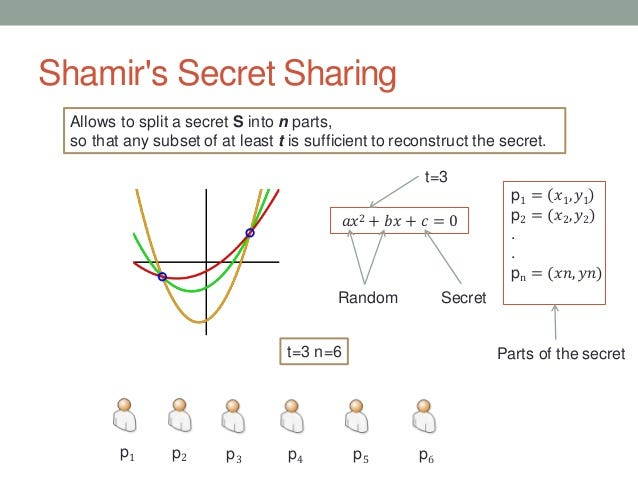STARK leverages this property and only reveals limited points on the derived polynomial in the proof step, thus ensuring the zero-knowledge requirement. In fact, since this method does not rely on a cryptographic function that can be broken (quantum computers), it is arguably safer.

## 6. Proof

Now, we have gone so far as transforming the initial program into a secured polynomial that contains (hopefully enough) information about the original function. The next step is to somehow show the Verifier with high confidence that the Prover has indeed done what it promised to do, with minimal chance for cheating.

## SNARK

To prove the validity of the polynomial equality `A * B — C = H * Z` (already encrypted through pairing) is true, SNARK Verifier does not directly plug in the solution `x` and checks if `A(x) * B(x) — C(x) = H(x) * Z(x)` is true. Not only because we want to save on the computation time of potentially thousands of terms of polynomials since we are already dealing with encrypted polynomial through elliptic curve pairing, but there are also a few tricks below that can be used to prove the equality requiring only minimal pieces of information from the prover side.

(all values below are encrypted using ECC)

1. For any of `A,B,C` , `A(x)` by definition is a linear combination of `A_1(t),A_2(t)...` where t is some arbitrary secret value. Using pairing checks, `A(x)` can be verified to be valid using a pre-generated list of `A_1(t),A_2(t)...`
2. The `x` used in `A,B,C` must be the same `x` , in other words, the Prover didn't massage the variables just so that it fits the equality. Using the same idea as above, this can be verified by checking if `A,B,C` is a linear combination of a pre-generated list of `A_i(t)+B_i(t)+C_i(t), 1<=i<= size(x)`
3. Once all the parameters are validated, `A(x) * B(x) — C(x) = H(x) * Z(x)` is checked at last. Given `A,B,C,H` generated by the Prover, and `Z` (easily) generated by the Verifier, a product check is trivial using pairing check

(step 1 below generates `Z` , everything is thus shifted down by 1)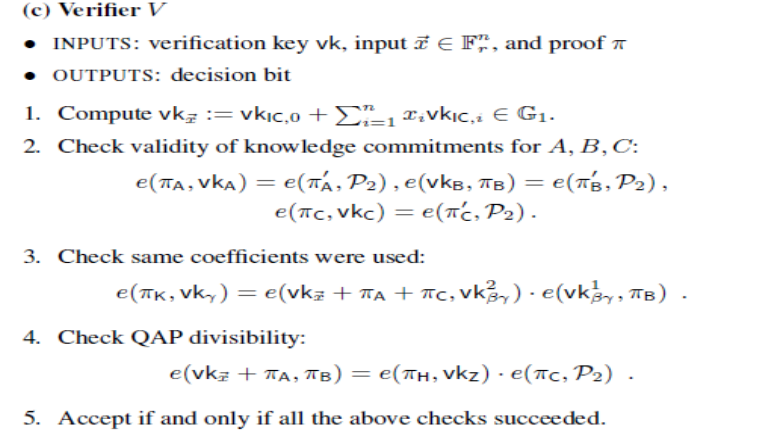STARK

Remember the two parts of the AIR we created? One is the execution trace, and one is the constraint system. Naturally, there is a relationship between the two (duh, the execution trace is the result of running the constraint system->code).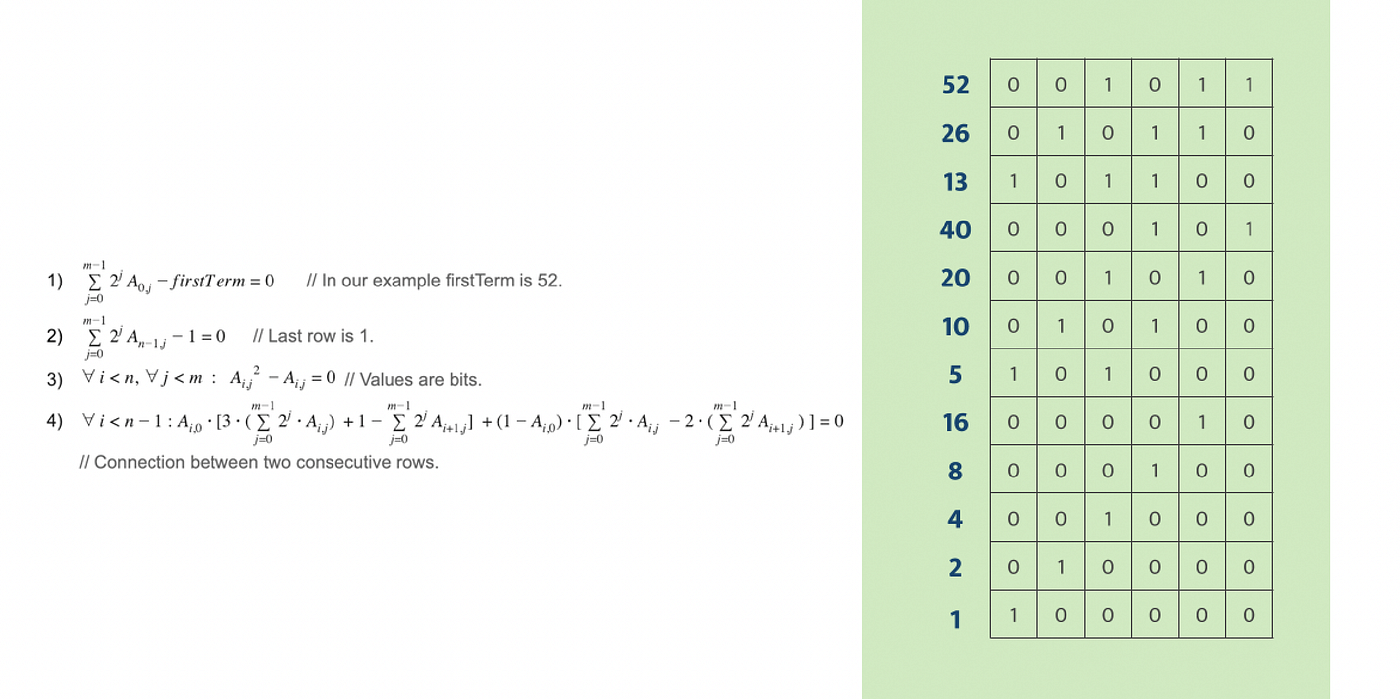From earlier

If we turn our constraint polynomial into the form `p(x)=0` , where the zeros of the function are are the constraint-compliant traces. Then, there is a special polynomial that can only be created if the execution trace properly reflects the changes specified by the constraints.

To illustrate, let's take the example of a simple polynomial `p(x)` . It can have iff some root `a` which means `(x-a)q(x)=p(x)` where `deg(p)=deg(q)+1` .Thus, `q(x)=p(x)/(x-a)` .

Then let's consider the case when `p` has `k` roots `a_0...a_(k-1)` then we have some polynomial of degree `deg(p)-k` equal to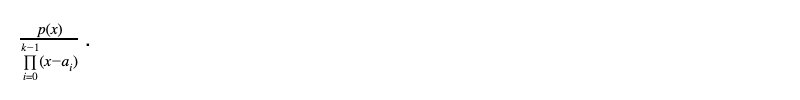This polynomial is only possible (no remainder) when the roots of `p` contains all `k` of `a_i`.

Now imagine the numerator is now a recurrence relation polynomial that encodes the problem. For Fibonacci, this would be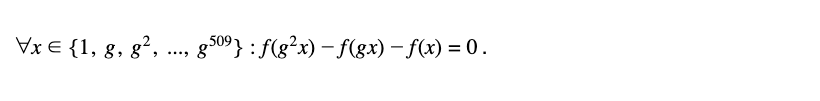Here the `g` or generator is used to index the inter-row relationship uniquely.

If we have the correct execution trace of the 510 steps that satisfy this relationship, then it's easy to reduce the dimension of the polynomial and create `q(x)` :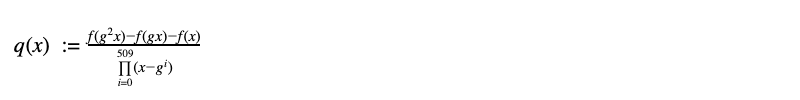As we proved above, this polynomial of reduced reduction exists iff you have the correct roots (in our case, also ordered). Thus, the presence of this polynomial is a certificate of the truthful execution of the program, as long as it satisfies:

1. the q(x) of low degree ( p(x) is < # of trace steps[because of interpolation], q= p-k)

Now we have transformed the proof into proving whether such a polynomial of low degree exists.

Proving a polynomial of low degree exists

Checking the claim of "a polynomial of a low degree" with 100% confidence needs a full check of all the `f(x)` over the entire domain, since it only takes one "bad" point to invalidate the statement.

However, it is possible to test whether a polynomial is sufficiently "close" to the claim by first sampling `d` points to construct an interpolated polynomial of dimension `d` , then a** testing point is randomly chosen to check if the polynomial is correct on the chosen point.** This test of proximity combined with the arithmetization step which augments the error is sufficiently accurate to determine a polynomial is indeed a low degree one.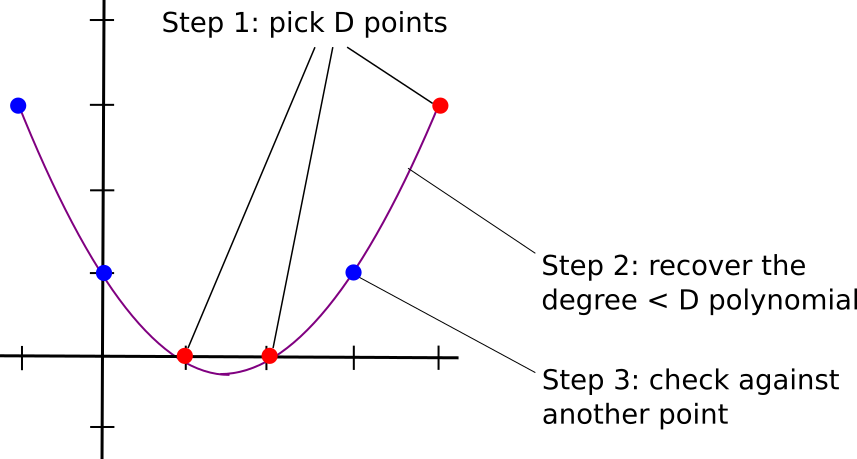This test takes d+1 samples, which is linear to the size of the computation trace. Of course STARK can do exponentially (literally) better.

FRI Protocol

Rather than performing the test above with `d+1`queries, STARK uses an FRI protocol which reduces the number of queries to O(log n).

For a given polynomial the Prover wants to prove and Verifier wants to verify, a randomness value in the domain is sent to the Prover, who needs to create

``````f₀(x)=g₀(x²)+xh₀(x²),
``````

essentially representing the original polynomial as a linear combination of two polynomials, each with half the `d` (each representing the even and odd terms). Then, a new polynomial f₁ is constructed with 𝛼₀ being a random value picked by the Verifier.

``````f₁(x)=g₀(x) + 𝛼₀h₀(x)
``````

f₁, being constructed by a linear combination of two `d/2` polynomials, is a `d/2` polynomial itself with extremely high probability.

At this point, we only need to prove that f₁ is indeed a `d/2` polynomial to prove that the original polynomial f₀ is a low degree polynomial.

By applying this kind of reduction recursively, the direct test can eventually be performed on a constant polynomial. It's easy to see that this kind of halfing the work operation gets us the desired O(log n) runtime.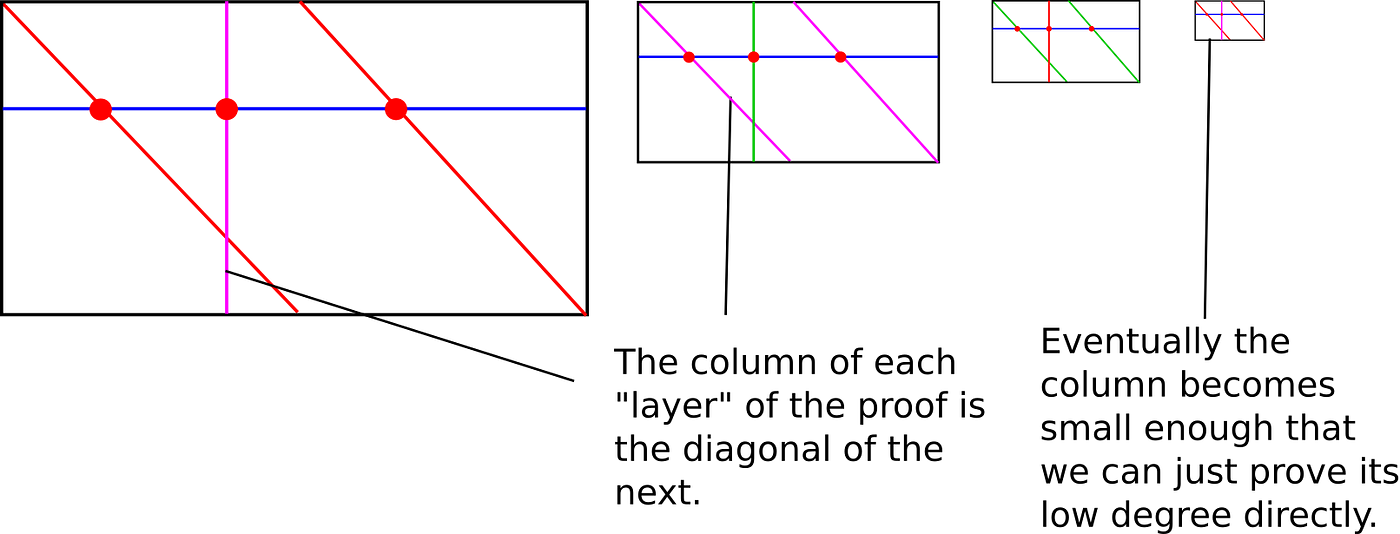Once all the generated work is wrapped up by the Prover and sent to the Verifier, it simply sends another random value `z`that checks all the polynomials at some random point and see if the results are correct.

In reality, the 𝛼 and `z`are not provided in an interactive model, instead, it is generated by using a hash chain of the intermediate steps, which is equally tamper-proof and ensures randomness by making sure the random value is only generated after the intended checking value is committed.

If all is verified, then the CI is proven.

## Summary

ZKP is arguably a revolutionary technology with its capability amplified by blockchain. Not only does it offer a potential path for off-chain scaling, but it also enables private computing with the **CI guarantee **— something that will empower much more than just blockchain.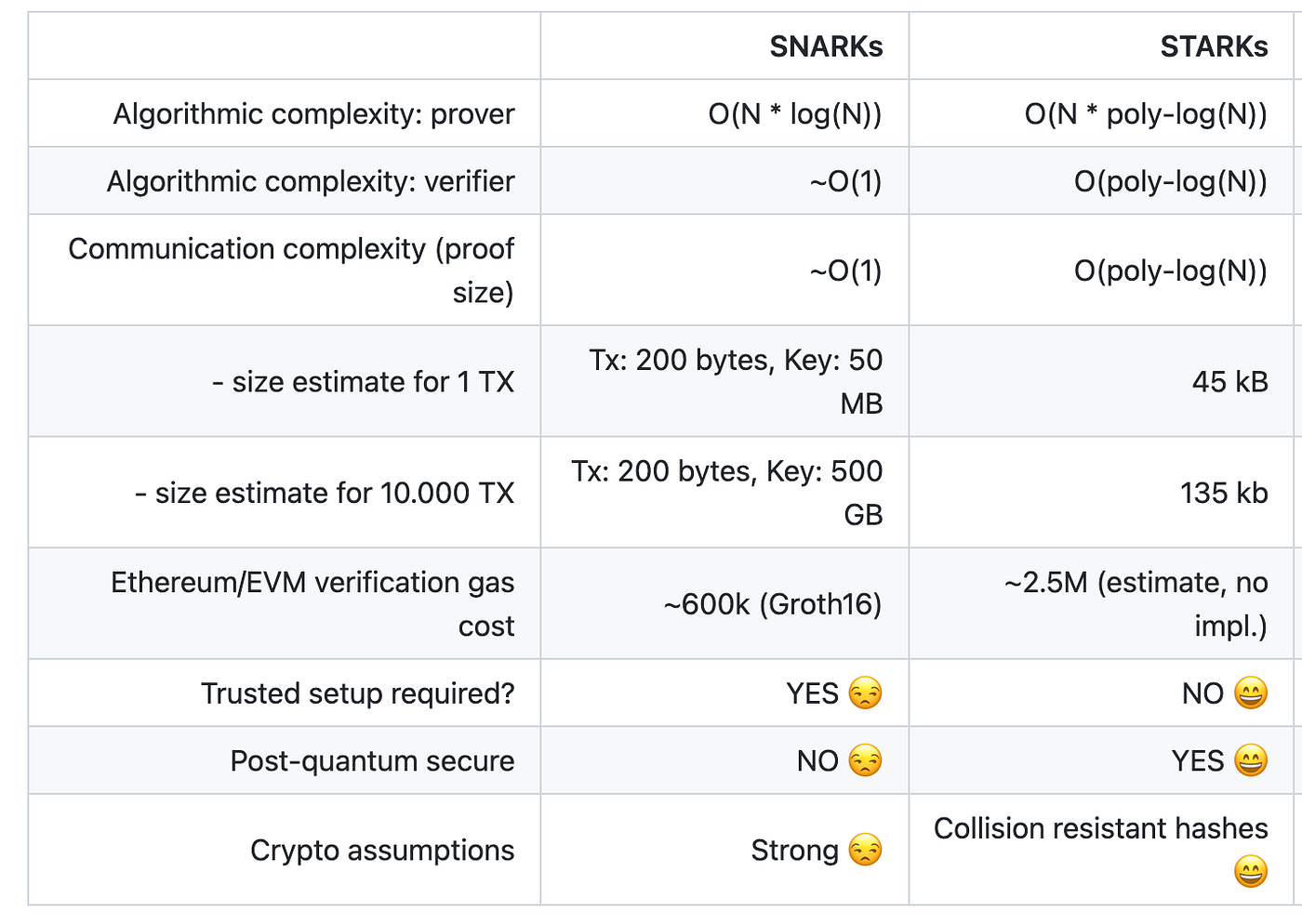As for SNARK and STARK, STARK is looking more promising with its low crypto assumption and scalable nature. However, the small proof size of SNARK can make it irreplaceable in certain applications. It's is still too early to call which one would be the winner. Perhaps, there would be a "multi-ZK" future.

I really hope you learned a bit more about how ZK works and no longer have to rely on the grossly simplified "it's magic" to trust ZK-enabled technology and application.

Let me know if there are any errors!